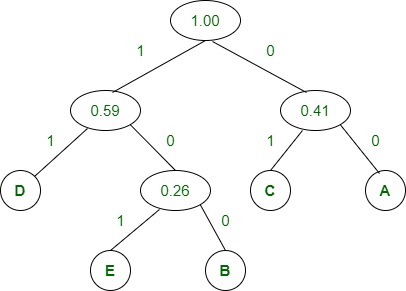Related Articles

# ISRO | ISRO CS 2020 | Question 19

• Last Updated : 03 Sep, 2020

Huffman tree is constructed for the following data: {A, B, C, D, E} with frequency {0.17, 0.11, 0.24, 0.33 and 0.15} respectively. 100 00 01101 is decoded as
(A) BACE

Explanation: Given,

```A = 0.17
B = 0.11
C = 0.24
D = 0.33
E = 0.15 ```

Now Huffman tree is,Hence, Huffman code:

```A : 00
B : 100
C : 01
D : 11
E : 101  ```

Therefore,

```= 100 00 01 101
= B A C E ```

Option (A) is correct.

Quiz of this Question

My Personal Notes arrow_drop_up